# 925 USD

Four classmates saved an annual total 925 USD. The second save twice as the first, third 35 USD more than the second and fourth 10 USD less than the first. How USD save each of them?

a =  150
b =  300
c =  335
d =  140

### Step-by-step explanation:

a+b+c+d=925
b=2a
c = 35+b
d = a-10

a+b+c+d=925
b=2•a
c = 35+b
d = a-10

a+b+c+d = 925
2a-b = 0
b-c = -35
a-d = 10

a = 150
b = 300
c = 335
d = 140

Our linear equations calculator calculates it.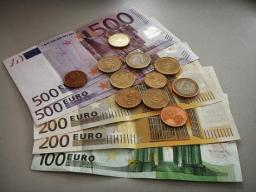Did you find an error or inaccuracy? Feel free to write us. Thank you!Tips to related online calculators
Do you have a system of equations and looking for calculator system of linear equations?

## Related math problems and questions:

• Gasoline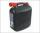35 l of gasoline is to be divided into four canisters so that in the third canister was 5 l less than the first canister, the fourth canister 10 l more than in the third canister and the second canister half what in the first canister. How many liters of
• RewardThree workers shared a common reward 13110 CZK: the first worker got 35% less than the second, and the third worker got 20% more than the second worker. How much got each worker?
• Gasoline canisters35 liters of gasoline is to be divided into 4 canisters so that in the third canister will have 5 liters less than in the first canister, the fourth canister 10 liters more than the third canister and the second canister half of that in the first canister
• Four pupilsFour pupils divided \$ 1485 so that the second received 50% less than the first, the third 1/2 less than a fourth, and fourth \$ 154 less than the first. How much money had each of them?
• Siblings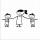Three siblings had saved up a total of 1,274 CZK. Peter had saved up to 15% more than Jirka and Hanka 10% less than Peter. How much money did they save each one of them?
• Boys and money270 USD boys divided so that Peter got three times more than Paul and Ivan has 120 USD more than Paul. How much each received?
• TrioTrio of workers earn 750 euros. Money divided by the work that each of them made​​. First received twice as the second, the second received three times more than the third. How many got everyone from workers?
• RepairingThree employees earned a total of € 469 for repairing the equipment. They split so that the first got 20% more than the second, and the third 15% more than the second. How many euros did everyone get?
• Savings 2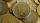Jozef and Michael saved 46 euros together. Michael saved 22 euros more than Jozef. How much did save each of them?
• RemunerationTotal remuneration for workers is 47 000 CZK. The second worker received 20% more than the first and third 4000 CZK less than a second employee. How many CZK each received?
• Part-time workersThree part-time workers received CZK 1,235 for their work. The first received 20% less than the second and the third received 45 CZK more than the second. Determine how many crowns (CZK) each of them received.
• Three workers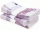The three workers received € 2,850 together for the work done. They divided them according to the time worked so that the first received 20% less than the second and the third € 50 more than the second. How much EUR did each worker receive?
• Three friends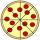Divide 570 euros to three friends so that first will get 50 euros less than the second and third twice more than the first. How many euros will get everyone?
• 3 masonsThree masons received 7,700 CZK. The second half received 1/2 more than the first and third twice more than the second mason. How much they each got crowns?
• ContestantsThe three best contestants are to divide the total prize of CZK 4,200. The second gets 20% more than the third. And the first one gets 200 CZK less than the second and the third together. How much will everyone get?
• EurosPeter, Jane, and Thomas have together € 550. Tomas has 20 euros more than Jane, Peter € 150 less than Thomas. Determine how much has each of them.
• Apartments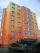Apartment on the first floor was 10% more expensive than the same apartment on the second floor. The difference was 105 Kc annually. Calculate the annual rent of the apartment in the first floor and from apartment on the second floor.